Courses

# Rotational Motion and Moment of Inertia Class 11 Notes | EduRev

## JEE : Rotational Motion and Moment of Inertia Class 11 Notes | EduRev

The document Rotational Motion and Moment of Inertia Class 11 Notes | EduRev is a part of the JEE Course Physics Class 11.
All you need of JEE at this link: JEE

Rotational Motion

1. Rigid Body

Rigid body is defined as a system of particles in which distance between each pair of particles remains constant (with respect to time) that means the shape and size do not change, during the motion. Eg. Fan, Pen, Table, stone and so on.

Our body is not a rigid body, two blocks with a spring attached between them is also not a rigid body. For every pair of particles in a rigid body, there is no velocity of seperation or approach between the particles. In the figure shown velocities of A and B with respect to ground are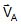and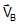respectively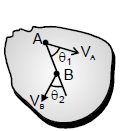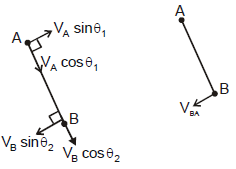If the above body Is rigid
VA cos θ1 = VB cos θ2

Note : With respect to any particle of rigid body the motion of any other particle of that rigid body is circular.

VBA = relative velocity of B with respect to A.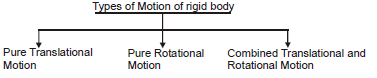1.1. Pure Translational Motion :

A body is said to be in pure translational motion if the displacement of each particle is same during any time interval however small or large. In this motion all the particles have same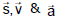at an instant.

example.

A box is being pushed on a horizontal surface.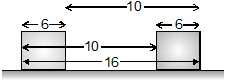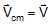of any particle,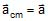of any particle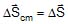of any particle

For pure translational motion :-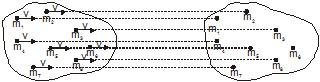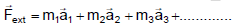Where m1, m2, m3, ......... are the masses of different particles of the body having accelerations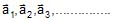respectively.

But acceleration of all the particles are same So,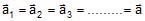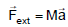Where M = Total mass of the body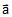= acceleration of any particle or of centre of mass of body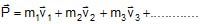Where m1, m2, m3 ...... are the masses of different particles of the body having velocities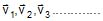respectively

But velocities of all the particles are same so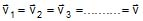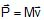Where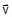= velocity of any particle or of centre of mass of the body.

Total Kinetic Energy of body =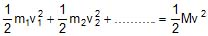1.2. Pure Rotational Motion :

A body is said to be in pure rotational motion if the perpendicular distance of each particle remains constant from a fixed line or point and do not move parallel to the line, and that line is known as axis of rotation. In this motion all the particles have same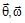and at an instant. Eg. : - a rotating ceiling fan, arms of a clock.

For pure rotation motion :-

Offer running on EduRev: Apply code STAYHOME200 to get INR 200 off on our premium plan EduRev Infinity!

## Physics Class 11

75 videos|295 docs|152 tests

,

,

,

,

,

,

,

,

,

,

,

,

,

,

,

,

,

,

,

,

,

;